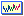luico_vlsi_06

# Quasi-Static Assignment of Voltages and Optional Cycles in Imprecise-Computation Systems with Energy Considerations

 Luis Alejandro Cortes ` ` Petru ElesZebo PengIEEE Trans. on Very Large Scale Integration Systems, Vol. 14, Issue 10, October 2006, pp. 1117-1129

ABSTRACT
For some realtime systems, it is possible to tradeoff precision for timeliness. For such systems, typically considered under the imprecise computation model, a function assigns reward to the application depending on the amount of computation allotted to it. Also, these systems often have stringent energy constraints since many such applications run on battery powered devices. We address in this paper, the problem of maximizing rewards for imprecise computation systems that have energy constraints, more specifically, the problem of determining the voltage at which each task runs as well as the number of optional cycles such that the total reward is maximal while time and energy constraints are satisfied. We propose a quasi-static approach that is able to exploit, with low online overhead, the dynamic slack that arises from variations in the actual number of task execution cycles. In our quasi-static approach, the problem is solved in two steps: first, at design off precision for timeliness. For such systems, considered typically under the Imprecise Computation model, a function assigns reward to the application depending on the amount of computation allotted to it. Also, many such applications run on battery-powered devices where the energy consumption is of utmost importance. We address in this paper the problem of energy minimization for Imprecise-Computation systems that have reward and time constraints. We propose a Quasi-Static (QS) approach that exploits, with low on-line overhead, the dynamic slack that arises from variations in the actual number of execution cycles: first, at design-time, a set of solutions are computed and stored (off-line phase); second, the selection among the precomputed assignments is left for run-time, based on actual values of time and reward (on-line phase).

Related files: